Latest Banking jobs   »   Quantitative Aptitude Quiz For RRB PO...

# Quantitative Aptitude Quiz For RRB PO Mains 2021- 2nd September

Direction (1 – 5):  In the given questions, two quantities are given, one as ‘Quantity I’ and another as ‘Quantity II’. You have to determine relationship between two quantities and choose the appropriate option:

Q1. Quantity I –Vessel A contains (Q + 36) liter mixture of milk & water in the ratio of 7 : 2, while vessel B contains (2Q + 42) liter mixture of milk & water in the ratio of 2 : 3. If 40% & 46% of mixture from vessel A and B taken out respectively, then remaining mixture in vessel B is 150% of remaining mixture in vessel A. Find the total initial quantity of milk in mixture of vessel A & vessel B together?
Quantity II –  Two vessels contains mixture of mango juice and orange juice in the ratio of 5 : 3 and 5 : 4 respectively. If 40 liter mixture from first vessel taken out and mixed in second vessel, so new ratio of mango juice and orange juice in second vessel becomes 25 : 19. Find initial quantity of mixture in second vessel?
(a) Quantity I > Quantity II
(b) Quantity I < Quantity II
(c) Quantity I ≥ Quantity II
(d) Quantity I ≤ Quantity II
(e) Quantity I = Quantity II or no relation

Q2. The ratio between radius of a sphere ball and a cylinder is 6 : 7 and that of between radius & height of cylinder is 7 : 4.
Quantity I – Ratio between volume of cylinder and volume of sphere ball.
Quantity II – Ratio between surface area of sphere and total surface area of cylinder.
(a) Quantity I > Quantity II
(b) Quantity I < Quantity II
(c) Quantity I ≥ Quantity II
(d) Quantity I ≤ Quantity II
(e) Quantity I = Quantity II or no relation

Q3. Quantity I – Center of semicircle B is O1 and radius is 28 cm. find area of shaded figure. BO1 is perpendicular to AC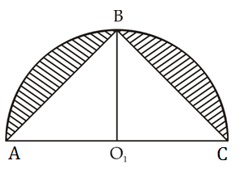Quantity II – Length of a rectangle is two times of side of square, while ratio between side of square to breadth of rectangle is 8 : 7. If perimeter of rectangle is 28 cm more than perimeter of square, then area of rectangle.
(a) Quantity I > Quantity II
(b) Quantity I < Quantity II
(c) Quantity I ≥ Quantity II
(d) Quantity I ≤ Quantity II
(e) Quantity I = Quantity II or no relation

Q4. Quantity I – Veer invested some amount in scheme A, which offers CI at 20% p.a., and equal amount in scheme B which offers SI at 25% pa. If difference between SI and CI received in 3rd year is Rs. 385, find sum invested in each scheme?
Quantity II – Veer, Sameer & Neeraj enter into a business by making investment in the ratio of 9 : 11 :13 respectively. If at the end of one year they got a total profit of Rs. 51150 Rs., then find the profit share of Neeraj?
(a) Quantity I > Quantity II
(b) Quantity I < Quantity II
(c) Quantity I ≥ Quantity II
(d) Quantity I ≤ Quantity II
(e) Quantity I = Quantity II or no relation

Q5. Quantity I – Coaching teacher asked a question to three students A, B & C and probability of question not being answered by three students is 0.5, 0.4, 0.7 respectively.  Find the probability that at most two students will solve the question.
Quantity II – A bag contains 5 green balls & 7 red balls, if three balls drawn at random from bag, then find probability of getting at least 1 green ball.
(a) Quantity I > Quantity II
(b) Quantity I < Quantity II
(c) Quantity I ≥ Quantity II
(d) Quantity I ≤ Quantity II
(e) Quantity I = Quantity II or no relation

Directions (6-10): Calculate quantity I and Quantity II on the basis of the given information then  compare them and answer the following questions accordingly.

Q6. Quantity I: How many days A would require to complete the work, working alone.
If two workers A and B working together completed a job in 5 days. If A worked twice as efficiently as he actually did and B worked 1/3 as efficiently as he actually did, the work would have been completed in 3 days.
Quantity II:  Arun completes 2/3 of a certain job in 6 days. Lakshya can complete 1/3 of the same job in 8 days and Pulkit can complete 3/4 of the work in 12 days. All of them work together for 4 days and then Arun and Pulkit quit. How long will it take for Lakshya to complete the remaining work alone?
(a) Quantity I > Quantity II
(b) Quantity I < Quantity II
(c) Quantity I ≥ Quantity II
(d) Quantity I ≤ Quantity II
(e) Quantity I = Quantity II

Q7. Quantity I:   A boat can travel ‘x’ km upstream in 4 hours and ‘y’ km downstream in 3 hours. If the speed of boat in still water is 5 km/h and the boat can travel a distance of ‘x+y’ km downstream in 4 hours, then find the speed of stream?
Quantity II:   If time taken to cover (D+11) km upstream is thrice the time taken to cover (D–3) km downstream. If ratio of speed of boat in upstream to the downstream is 3 : 7 and time taken to cover (D+18) km downstream is 2.5 hours what will be the speed of current?
(a) Quantity I > Quantity II
(b) Quantity I < Quantity II
(c) Quantity I ≥ Quantity II
(d) Quantity I ≤ Quantity II
(e) Quantity I = Quantity II

Q8. Quantity I:   The respective ratio between the present ages of son, mother, father and grandfather is 2 : 7 : 8  : 12. The average age of son and mother is 27 yr. What will be mother’s age after 7 yr?
Quantity II:  The age of the father 5 years ago was 5 times the age of his son. At present the father’s age is 3 times that of his son. What is the present age of the father ?
(a) Quantity I > Quantity II
(b) Quantity I < Quantity II
(c) Quantity I ≥ Quantity II
(d) Quantity I ≤ Quantity II
(e) Quantity I = Quantity II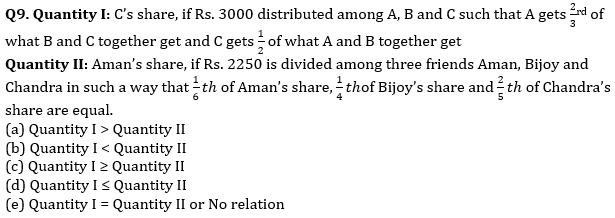Q10. A boat sails down the river for 10 km and then up the river for 6 km. The speed of the river is 1 km/hr.
Quantity I: Speed of boat in still water, if for the whole trip it takes 4 hours.
Quantity II: 4 km/hr
(a) Quantity I > Quantity II
(b) Quantity I < Quantity II
(c) Quantity I ≥ Quantity II
(d) Quantity I ≤ Quantity II
(e) Quantity I = Quantity II or No relation

Solutions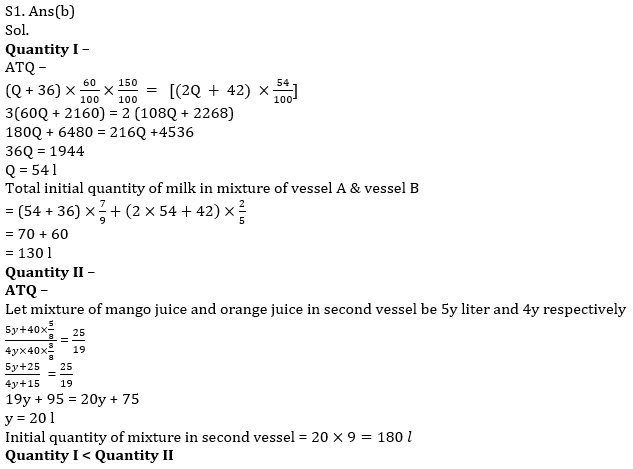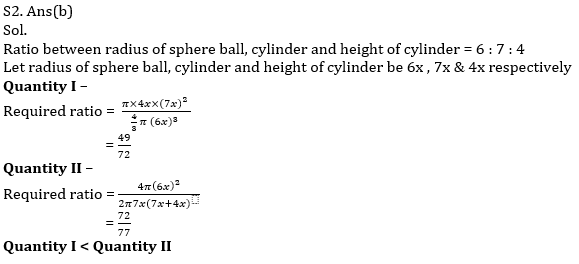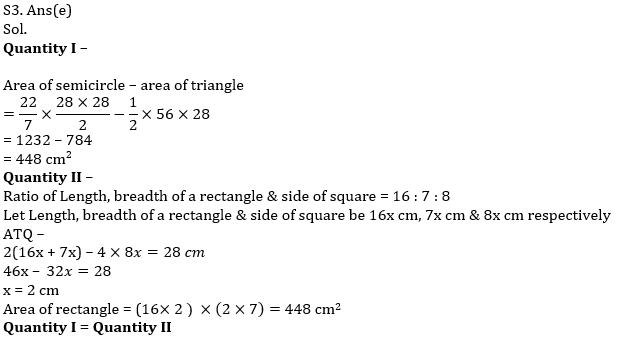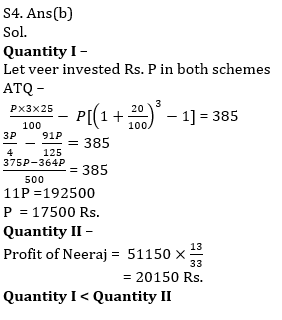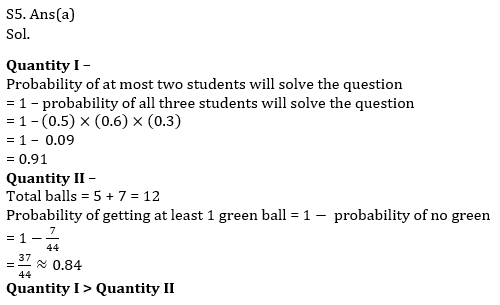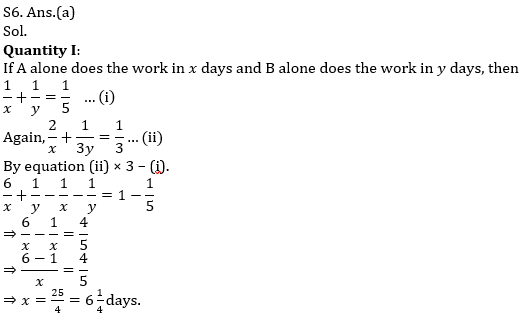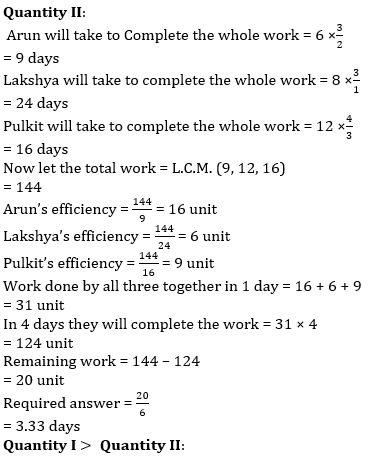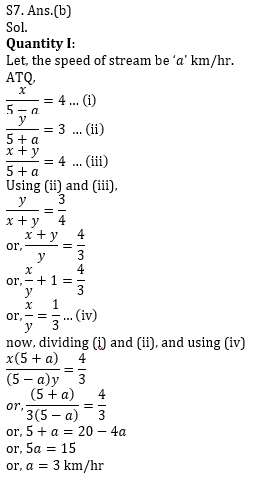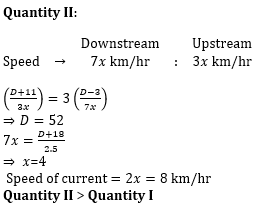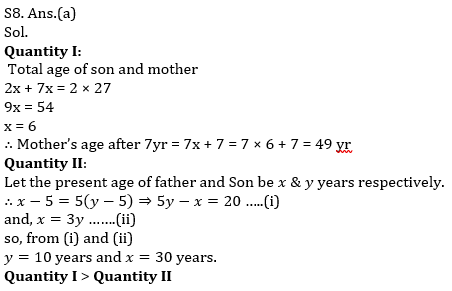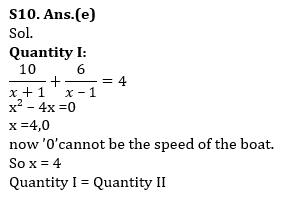Click Here to Register for Bank Exams 2021 Preparation Material#### Congratulations!Download Hindu Review of October 2021: Free PDF